# How to Calculate and Solve for Separator Gas Gravity in a Fluid | The Calculator Encyclopedia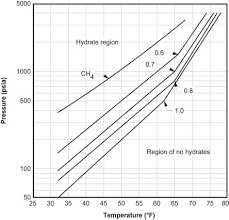The image above represents separator gas gravity.

To compute for the separator gas gravity, four essential parameters are needed and these parameters are API Gravity (°API), Actual Separator Temperature (°Rankine) (Tsep), Actual Separator Pressure (Psep) and Gas Gravity at Actual Separator Psep and Tsepg).

The formula for calculating the separator gas gravity:

γgs = γg[1 + 5.912(10-5)(°API)(Tsep – 460)log(Psep / 114.7)]

Where:

γgs = Separator Gas Gravity
°API = API Gravity
Tsep = Actual Separator Temperature (°Rankine)
Psep = Actual Separator Pressure
γg = Gas Gravity at Actual Separator Psep and Tsep

Let’s solve an example;
Find the separator gas gravity with an API Gravity of 21, actual separator temperature of 18, actual separator pressure of 14 and gas gravity at actual separator of 32.

This implies that;

°API = API Gravity = 21
Tsep = Actual Separator Temperature (°Rankine) = 18
Psep = Actual Separator Pressure = 14
γg = Gas Gravity at Actual Separator Psep and Tsep = 32

γgs = γg[1 + 5.912(10-5)(°API)(Tsep – 460)log(Psep / 114.7)]
γgs = 32[1 + 5.912(10-5)(21)(18 – 460)log(14 / 114.7)]
γgs = 32 [1 + 5.912(10-5)(21)(18 – 460) log(0.1220)]
γgs = 32 [1 + 5.912(10-5)(21)(-442) log(0.1220)]
γgs = 32 [1 + 5.912(10-5)(21) (-442) (-0.9134)]
γgs = 32 [1 + 0.501]
γgs = 32 [1.501]
γgs = 48.039

Therefore, the separator gas gravity is 48.039.

Nickzom Calculator – The Calculator Encyclopedia is capable of calculating the separator gas gravity.

To get the answer and workings of the separator gas gravity using the Nickzom Calculator – The Calculator Encyclopedia. First, you need to obtain the app.

You can get this app via any of these means:

To get access to the professional version via web, you need to register and subscribe for NGN 1,500 per annum to have utter access to all functionalities.
You can also try the demo version via https://www.nickzom.org/calculator

Apple (Paid) – https://itunes.apple.com/us/app/nickzom-calculator/id1331162702?mt=8
Once, you have obtained the calculator encyclopedia app, proceed to the Calculator Map, then click on Petroleum under EngineeringNow, Click on Fluid Properties under Petroleum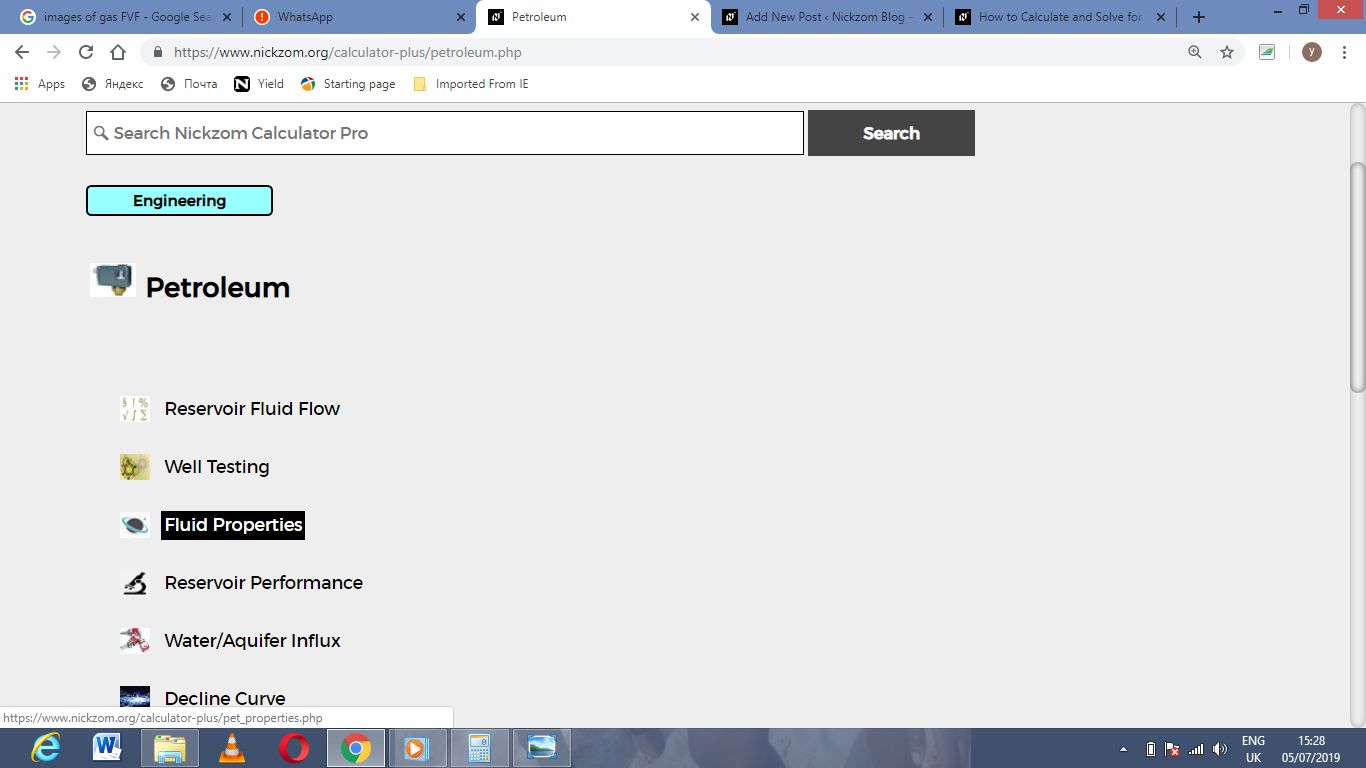Now, Click on Separator Gas Gravity under Fluid PropertiesThe screenshot below displays the page or activity to enter your values, to get the answer for the separator gas gravity according to the respective parameters which are the API Gravity (°API), Actual Separator Temperature (°Rankine) (Tsep), Actual Separator Pressure (Psep) and Gas Gravity at Actual Separator Psep and Tsepg).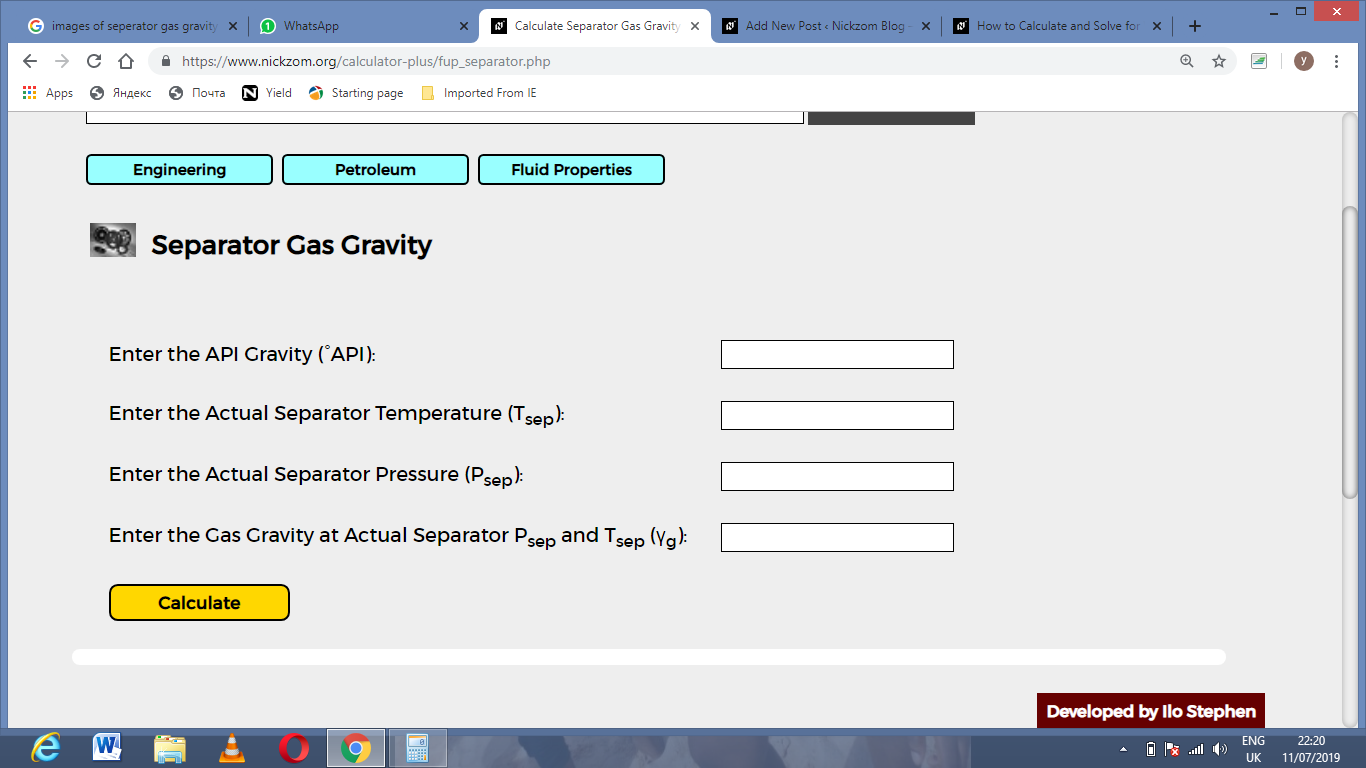Now, enter the values appropriately and accordingly for the parameters as required by the API Gravity (°API) is 21, Actual Separator Temperature (°Rankine) (Tsep) is 18, Actual Separator Pressure (Psep) is 14 and Gas Gravity at Actual Separator Psep and Tsepg) is 32.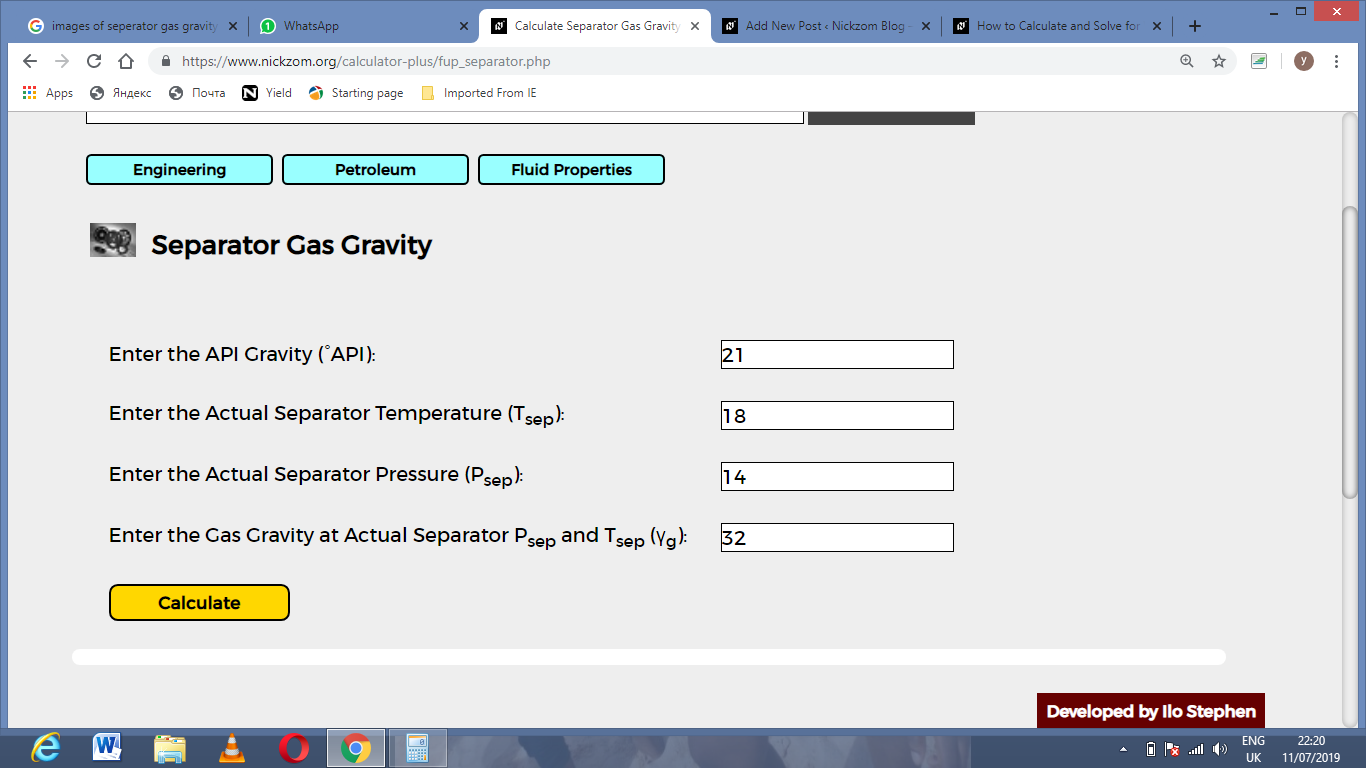Finally, Click on Calculate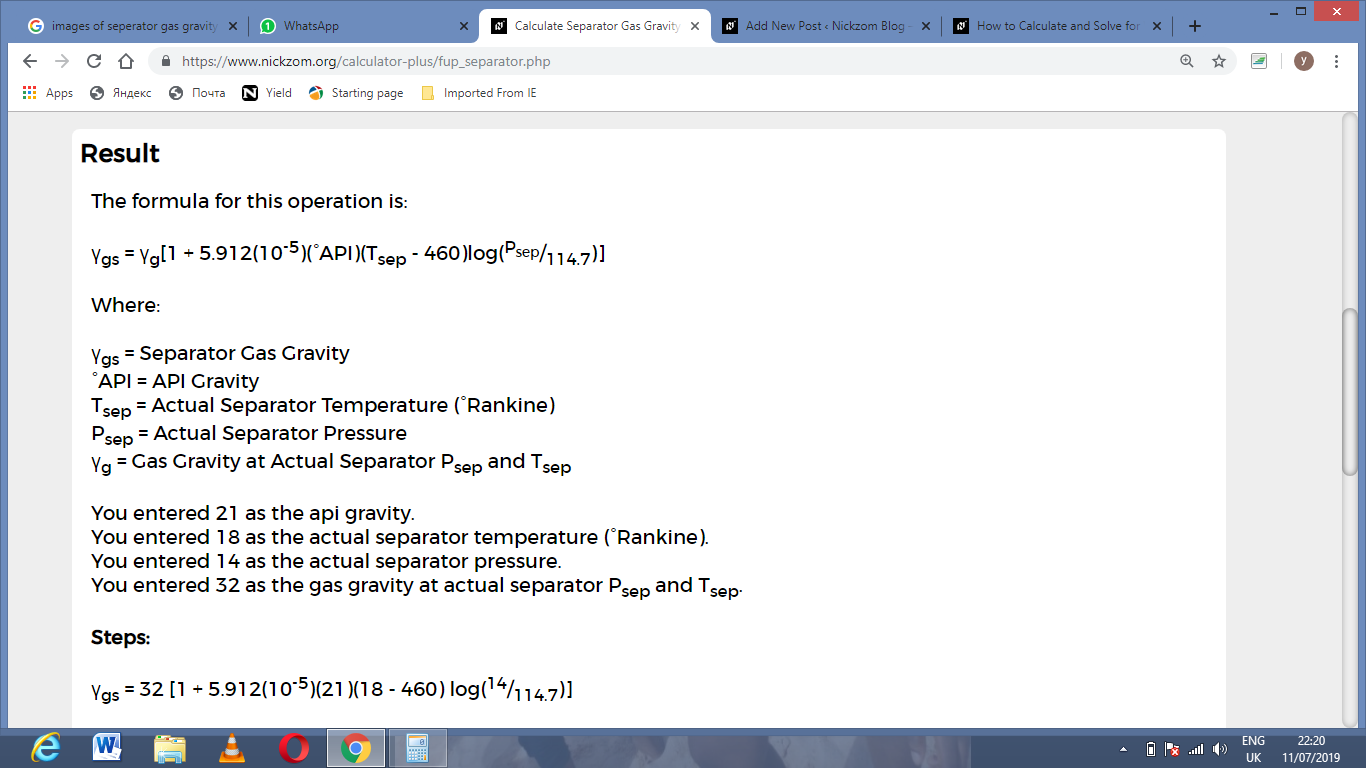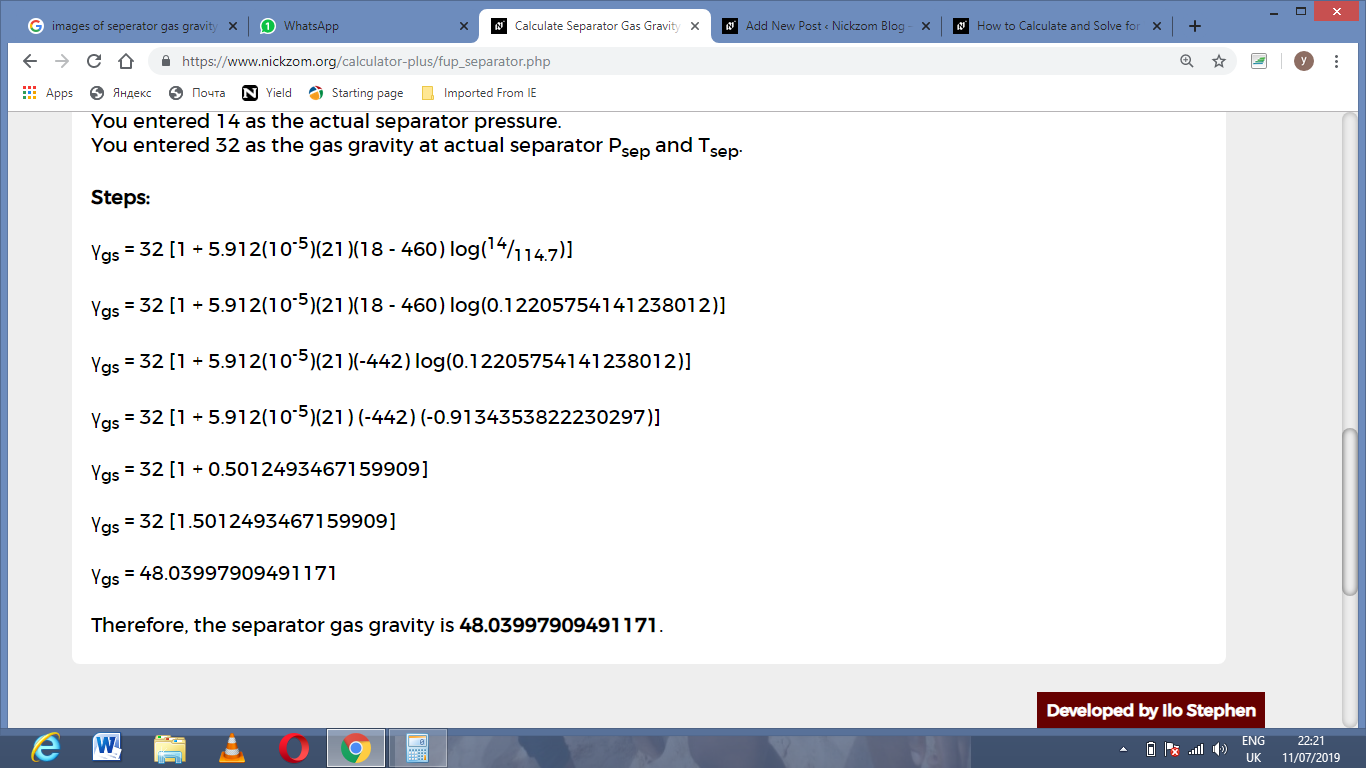As you can see from the screenshot above, Nickzom Calculator– The Calculator Encyclopedia solves for the separator gas gravity and presents the formula, workings and steps too.